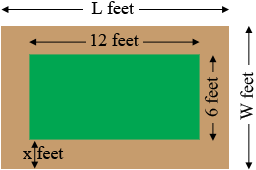SEARCH HOMEMath Central Quandaries & QueriesQuestion from Abigail, a student: A garden measures 12 feet by 16 feet. The family would like to add a stone walkway around the garden. This would increase the total area of to 302.25 square feet. what will be the width of the of the walkwayHi Abigail,

I drew a diagram with the walkway $x$ feet wide, all the way around the garden.In my diagram the length of the garden plus the walkway is $L$ feet and its width is $W$ feet. Write $L$ and $W$ in terms of $x.$ $L \times W = 302.25$ square feet. Solve for $x.$

PennyMath Central is supported by the University of Regina and the Imperial Oil Foundation.